# Tea blend

Tea blends are maked from two kinds of tea. In standard tea mixture are two teas in the ratio 1:3 and 40 g costs 42 CZK. In the premium tea mixture are weighing two teas in the ratio 1:1 and 50 grams costs 60 CZK. How much cost 10 grams of more expensive kind of tea?

Correct result:

x =  15

#### Solution:

(40/10)(1x+3y)/(1+3)=42
(50/10)(x+y)/(1+1) = 60

(40/10)•(1•x+3•y)/(1+3)=42
(50/10)•(x+y)/(1+1) = 60

10x+30y = 420
25x+25y = 600

x = 15
y = 9

Our linear equations calculator calculates it.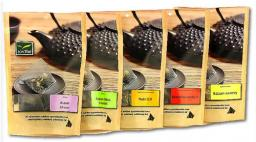We would be pleased if you find an error in the word problem, spelling mistakes, or inaccuracies and send it to us. Thank you!Tips to related online calculators
Check out our ratio calculator.
Do you have a system of equations and looking for calculator system of linear equations?

## Next similar math problems:

• Two teas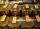A mixture weighing 10 kg was made from two types of tea. The price of tea was 160 CZK/kg and second tea 170 CZK/kg. The price of the mixture is 166 CZK/kg. How many kilograms of each type of tea had to be mixed?
• CandyThe price for 1kg of more expensive candy is 125 CZK. The price for 1kg of cheaper candy is 100 CZK. We make two different blends of candy. And now. The second blend contains 2kg of more expensive candy and several kg of cheaper candy. The price per 1 kg
• Coffee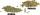Coffee merchant has coffee robusta and arabica species. 1 kg Robusta worth 450 CZK, Arabica 1 kg is 300 CZK more expensive. Calculate how many kilograms of Robusta and Arabica will need to produce 30 kg of the mixture so that the mixture cost is 490 CZK p
• CoffeeIn stock are three kinds of branded coffee prices: I. kind......205 Kc/kg II. kind......274 Kc/kg III. kind.....168 Kc/kg Mixing these three species in the ratio 8:5:6 create a mixture. What will be the price of 100 grams of this mixture?
• MixingIf we mix 5 kg of goods of one kind and 3 kg second one, resulting mixture cost 16.50 EUR/kg. If these quantities are mixed in reverse - first three kilograms and 5 kilograms second cost of mixture is 18.50 EUR/kg. What is the price of one kg of goods of
• SweetsWe want to prepare 5 kg of sweets for 150 CZK. We will mix cheaper candy: 1 kg for 120 CZK and more expensive candy: 1 kg per 240 CZK. How much of this two types of candy is necessary to prepare this mixture?
• Candies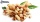In the confectionery, the price for 1 kg of pistachio candies cost CZK 360, and the price for 1 kg of hazelnut candies was CZK 280. Mixing these two types of sweets created a box of chocolates. How many grams of pistachios and how many grams of hazelnut c
• Two wine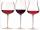In 2:1 mix wine solution cost 4.1 USD. In 1:2 mix wine solution cost 4.5 USD. How much cost liter of each wine?
• Tea mixture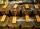Of the two sort of tea at a price of 180 CZK/kg and 240 CZK/kg we make a mixture 12 kg that should be prepared at a price of 200 CZK / kg. How many kilos of each sort of tea will we need to be mixed?
• CandiesThe price for 1 kg of more expensive candies is 125 CZK. The price for 1 kg of cheaper candy is 100 CZK. We mix two different mixtures of candy. A) The first mixture contains 2 kg more expensive and 0.5 kg cheaper candy. Calculate the price for 1 kg of th
• WineA bottle of wine costs 21 euros; and wine is 20 times more expensive than a bottle. How much a bottle cost?
• Alcohol mixtureFrom 55% and 80% alcohol we have to produce 0.2 kg of 60% alcohol. How many of them do we use in solution?
• ConcentrationHow many grams of 65% and 50% acid is to be mixed to give 240 g acid with a concentration of 60%?
• CandiesThere are two types of candies in the store at 20 CZK/kg and 80 CZK/kg. How much will be cost a mix if we mix 22kg cheaper and 4kg more expensive candies?
• Bottle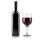Bottle with wine costs 58 USD. Wine is 27 USD more expensive than the empty bottle. How much is the bottle itself?
• Bonbons 2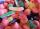Kilo sweets will cost 260 CZK. The first type has a price per 320 kg, the second type 240 CZK per kg. How many kilos of both kinds of sweets need to prepare a 100 kg mixture ?
• Mixture of nutsThe mixture of nuts should be prepared from almonds, peanuts and cashew nuts ratio 1: 2: 3 (respectively). The price of almonds is 150 CZK/kg, the price of peanuts is 140 CZK/kg and the price of cashew nuts is 180 CZK/kg. The price of the mixture is deter